Important Questions: Quadratic Equations

# Important Questions: Quadratic Equations

Test Description

## 10 Questions MCQ Test Mathematics (Maths) Class 10 | Important Questions: Quadratic Equations

Important Questions: Quadratic Equations for Class 10 2022 is part of Mathematics (Maths) Class 10 preparation. The Important Questions: Quadratic Equations questions and answers have been prepared according to the Class 10 exam syllabus.The Important Questions: Quadratic Equations MCQs are made for Class 10 2022 Exam. Find important definitions, questions, notes, meanings, examples, exercises, MCQs and online tests for Important Questions: Quadratic Equations below.
Solutions of Important Questions: Quadratic Equations questions in English are available as part of our Mathematics (Maths) Class 10 for Class 10 & Important Questions: Quadratic Equations solutions in Hindi for Mathematics (Maths) Class 10 course. Download more important topics, notes, lectures and mock test series for Class 10 Exam by signing up for free. Attempt Important Questions: Quadratic Equations | 10 questions in 10 minutes | Mock test for Class 10 preparation | Free important questions MCQ to study Mathematics (Maths) Class 10 for Class 10 Exam | Download free PDF with solutions
 1 Crore+ students have signed up on EduRev. Have you?
Important Questions: Quadratic Equations - Question 1

### Which of the following is a quadratic equation?

Detailed Solution for Important Questions: Quadratic Equations - Question 1

The correct answer is d

A quadratic equation (from the Latin quadratus for "square") is any equation that can be rearranged in standard form as. where x represents an unknown, and a, b, and c represent known numbers, where a ≠ 0. If a = 0, then the equation is linear, not quadratic, as there is no. term.

Therefore, x3 - x2 = ( x -1)3 is a quadratic equation.

Important Questions: Quadratic Equations - Question 2

### Which of the following is not a quadratic equation?

Detailed Solution for Important Questions: Quadratic Equations - Question 2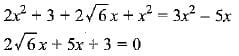Important Questions: Quadratic Equations - Question 3

### Which of the following equations has 2 as a root?

Detailed Solution for Important Questions: Quadratic Equations - Question 3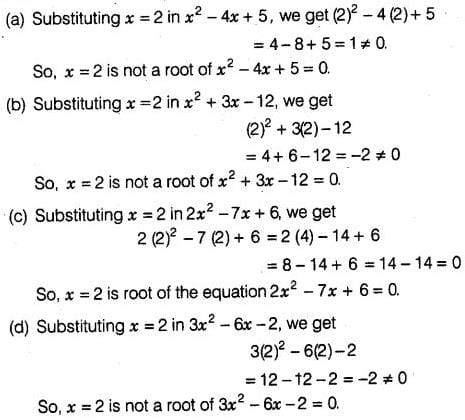Important Questions: Quadratic Equations - Question 4

Which of the following are the roots of the quadratic equation, x2 - 9x + 20 = 0 by factorisation?

Detailed Solution for Important Questions: Quadratic Equations - Question 4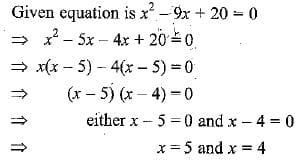∴ x = 4 and 5 are the roots/solution of the given quadratic equation

Important Questions: Quadratic Equations - Question 5

Value(s) of k for which the quadratic equation 2x2 -kx + k = 0 has equal roots is

Important Questions: Quadratic Equations - Question 6

If p,q and r are rational numbers and p ≠ q ≠ r, then roots of the equation (p2 - q2)x2 - (q2 - r2)x + (r2 - p2) = 0 are

Detailed Solution for Important Questions: Quadratic Equations - Question 6

Putting x = - 1, we have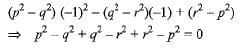∴ x = - 1 is one root.
Only option (d) has one root - 1.

Important Questions: Quadratic Equations - Question 7

The two consecutive odd positive integers, sum of whose squares is 290 are

Important Questions: Quadratic Equations - Question 8

If α, β are roots of the equation x2 + 5x + 5 = 0, then equation whose roots are α + 1 and β + 1 is

Detailed Solution for Important Questions: Quadratic Equations - Question 8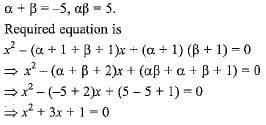Important Questions: Quadratic Equations - Question 9

The quadratic equation with real co-efficients whose one root is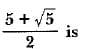Important Questions: Quadratic Equations - Question 10

Which of the following equations has two distinct real roots ?

## Mathematics (Maths) Class 10

53 videos|369 docs|138 tests
 Use Code STAYHOME200 and get INR 200 additional OFF Use Coupon Code
Information about Important Questions: Quadratic Equations Page
In this test you can find the Exam questions for Important Questions: Quadratic Equations solved & explained in the simplest way possible. Besides giving Questions and answers for Important Questions: Quadratic Equations, EduRev gives you an ample number of Online tests for practice

## Mathematics (Maths) Class 10

53 videos|369 docs|138 tests